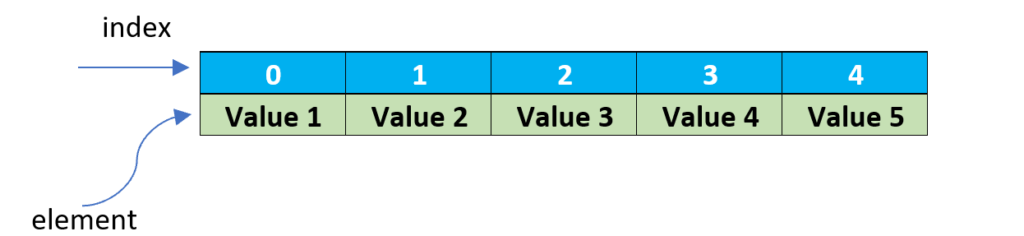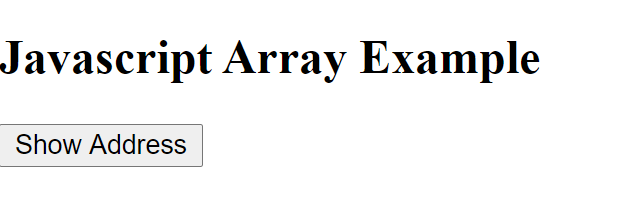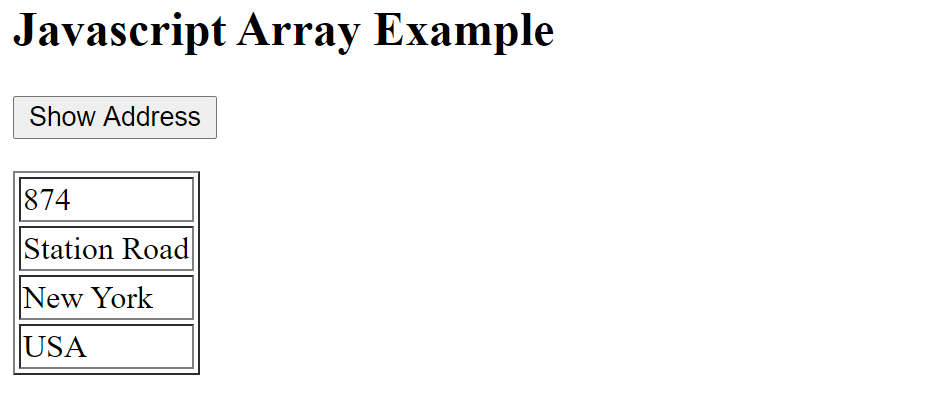# Array in Javascript

Array is an object in Javascript which is used to store the collection of values. Each value in the array is called as element. The elements can be different data type in the same array. The position of each element is called as index. The index is starts from 0.Javascript Array example

## Create array in Javascript

There are two ways to create array in Javascript. Let look into that with syntax.

### Array literal notation ([ ])

The easiest way to create an array is with an array literal, which is simply a comma separated list of array elements within square brackets

### Array() constructor

Another way to create array is using the Array( ) constructor. To do that, we need to call the constructor using new Array() with any of the following options.

• If we call the constructor without argument, it defines the empty array in Javascript
• If we call the constructor with single numeric argument, it specifies the length of the array.
• The elements can be passed as arguments in the Array() constructor.

### Example 1:

In this example, we are trying to store the student names in Javascript array. Here we named the variable as student and called the array constructor with the arguments of student names.

### Example 2 :

In this example, first we created the empty array variable named as student. Then we used one of the array method push( ) to add each name in the array.

OutputCreate array in Javascript

## Iterate over an array in Javascript

Like other programming languages (C,C++,Java and Python), We can use the for loop to iterate the elements of array in Javascript. In this example, the variable i is used as array index. As we defined i =0,the index position starts from 0. Also the variable student is the array which contains all the values.

Output

Kevin
Larry
Stephen
Alex

## Print Javascript array in HTML page

Lets create simple HTML page to show the value of array in the browser.Iterate elements of array in js

The above HTML page has the button “Show Address”. If we clicks that button, it will show the value of array in the same page. Here the array variable is address which contains the collection of values such as Door no, Street, State and Country details.

OutputDisplay array elements in HTML